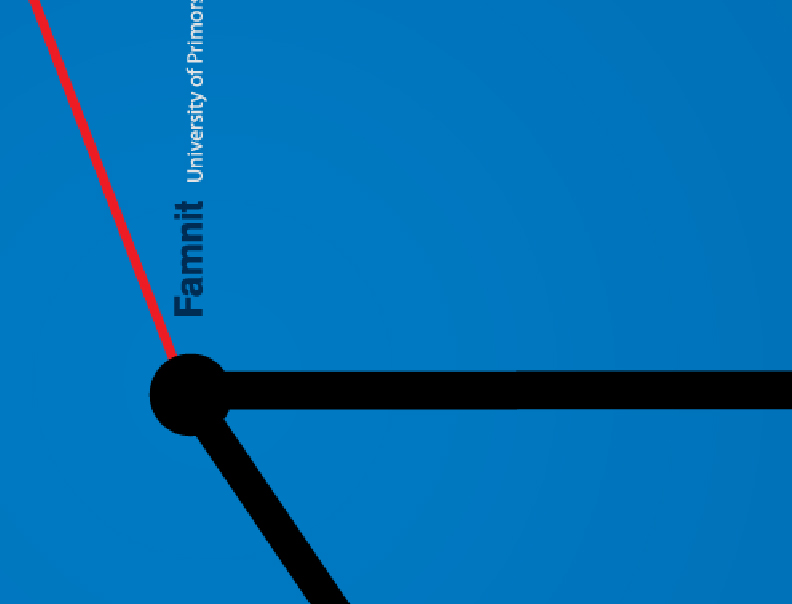Univerza na Primorskem Fakulteta za matematiko, naravoslovje in informacijske tehnologijeSI | EN# Raziskovalni matematični seminar - Arhiv

 2021 2020 2019 2018 2017 2016 2015 2014 2013 2012 2011 2010
 1 2 3 4 5 6 7 8 9 10 11 12
Datum in ura / Date and time: 2.12.13
(10:00-11:00)
Predavalnica / Location: FAMNIT-POŠTA
Predavatelj / Lecturer: Sergio Hiroki Koike Quintanar
Naslov / Title: On the isomorphism problem of cyclic Haar graphs

Datum in ura / Date and time: 25.11.13
(10:00-11:00)
Predavalnica / Location: FAMNIT-SEMIN
Predavatelj / Lecturer: prof. dr. Primož Potočnik (UL FMF and UP IAM)
Naslov / Title: How many automorphisms can a graph have?
Vsebina / Abstract:

The topic of the talk will be finite connected graphs admitting an automorphism group that acts transitively on the arcs of the graph. These graphs are traditionally called symmetric. The main theme of this talk is the following question:

How many automorphisms can a symmetric graph of order $n$ have?

For $n\geq 3$, the number of automorphisms of a symmetric graph on $n$ verticesis at least $2n$ and at most $n!$. (These values are sharp, as witnessed by cycles and complete graphs.)

The story becomes more interesting if one fixes the valence. Clearly, the number of automorphisms of a symmetric graphof valence $k$ and order $n$ is at least $kn$ (since $kn$ is the number of arcs), and at most $nk\sqrt{(k-1)!}^{\,n-1}$ (as can be shown without much trouble).

While this upper bound is quite good for some values of $k$, it is very far frombeing sharp for some others. For example, for every even $n$ at least $6$,one can construct a $4$-valent symmetric graph of order $n$ with $n\sqrt{2}^n$ automorphisms,showing that the above bound is almost sharp'' for $k=4$. Similar examples can be constructed for any composite $k$.

On the other hand, a classical result of Tutte says that a $3$-valent symmetric graph of order $n$ can have at most $48n$ automorphisms, and a similar linear bound can be proved for any prime value of $k$.

The question: under what circumstances the order of the automorphism group of a symmetric graph canbe bounded by a linear function of the order of the graph?'' is related to several classical problems, like the Sims conjecture on primitive groups,the Weiss conjecture on locally primitive graphs, or the Praeger conjecture on locally quasiprimitive graphs.

On the other hand, not much work has been done on determining which classes of symmetric graphs admit a polynomial (or even sub-exponential) bound on the order of their automorphism groups. Some recent results along these lines will be presented in the talk.

Datum in ura / Date and time: 18.11.13
(10:00-11:00)
Predavalnica / Location: FAMNIT-SEMIN
Predavatelj / Lecturer: Ratko Darda
Naslov / Title: Bounds on the Product Irregularity Strength of Graphs
Vsebina / Abstract:

For a graph X with at most one isolated vertex and without isolated edges, a product irregular labeling, is a labeling of its edges with the values from {1,2,...,s} in such a way that for any two distinct vertices u and v of X the product of labels of the edges incident with u is different from the product of labels of the edges incident with vThe minimal s for which there exist a product irregular labeling is called the product irregularity strength of X and is denoted by ps(X).

In this talk I will prove that  ps(X) ≤ |V(X)|-1, for any graph X with more than 3 vertices. I will also determine the product irregularity strength of the complete multipartite graphs.

This is a joint work with Ademir Hujdurović.

Datum in ura / Date and time: 11.11.13
(10:00-11:00)
Predavalnica / Location: FAMNIT-SEMIN
Predavatelj / Lecturer: Anja Komatar
Naslov / Title: Second Neighbourhood Conjecture
Vsebina / Abstract:

Second Neighbourhood Conjecture states that every oriented graph has a vertex with second neighbourhood at least as large as first. We show that if a counterexample exists, it has a large minimal degree and is strongly connected.